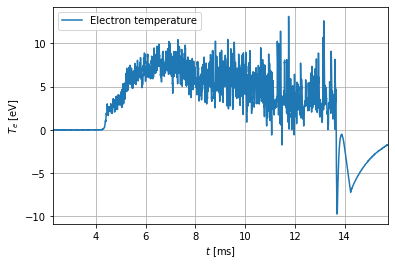# Electron temperature measurement with the ball-pen and Langmuir probe¶

The combined probe head (ball-pen probe + Langmuir probe) offers measurements of the plasma potential $\Phi$, floating potential $V_{fl}$ and electron temperature $T_e$ with high temporal resolution (1 $\mu$s). In this notebook, its data is loaded, processed and plotted.

Let's load some basic modules: numpy (for basic operations with arrays) and pylab (for plotting).

In :
# Import basic modules
import numpy as np
import pylab as pl
%matplotlib inline


## Basic data access¶

To access the GOLEM data, we write the function get_data.

In :
from urllib.error import HTTPError # recognise the error stemming from missing data
import pandas as pd # for reading csv files

#Define an exception which will be raised if the data is missing and stop the notebook execution
class StopExecution(Exception):
def _render_traceback_(self):
pass

def get_data(identifier, shot):
URL = "http://golem.fjfi.cvut.cz/shots/{shot}/Diagnostics/PetiProbe/{identifier}.csv"
url = URL.format(shot=shot, identifier=identifier)
try:
df = pd.read_csv(url, names=['time', identifier], index_col='time')
except HTTPError:
raise StopExecution
t = np.array(df.index)
data = np.transpose(np.array(df))
return t, data


To test get_data, we load and plot the Langmuir probe data from the current discharge. The signal is called U_LP.

In :
shot = 0
t, data = get_data('U_fl_LP', shot)
pl.plot(t*1000, data)
pl.xlabel('$t$ [ms]')
pl.ylabel('$V_{fl}$ [V]')
pl.grid(True)
pl.xlim(0,20)

Out:
(0, 20)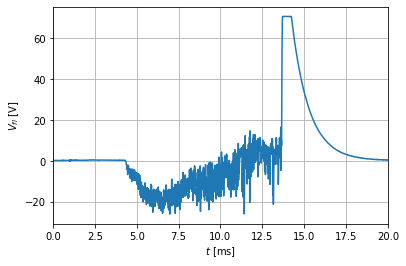Notice that we multiply the time axis by a factor of 1000 in the argument of the plot function and then plot it in miliseconds, which yields nicer numbers on the X axis.

## Discharge duration¶

In the following, we define the function shot_time(shot) which loads the times of the discharge beginning and end.

In :
from urllib.request import urlopen

def get_time(phase, shot):
url = 'http://golem.fjfi.cvut.cz/shots/%i/Diagnostics/BasicDiagnostics/t_plasma_%s' % (shot, phase)
f = urlopen(url)
f.close()
return float(data)/1000

def shot_time(shot):
'''Return edges of time of shot in ms.'''
return get_time('start', shot), get_time('end', shot)

t1, t2 = shot_time(shot)
print('The discharge lasted from %.1f ms to %.1f ms.' % (t1*1000, t2*1000))

The discharge lasted from 4.3 ms to 13.7 ms.


## Offset removal¶

An offset is a non-physical contribution to the signal, created by parasitic voltages, cross-talk between diagnostics, electronic noise and many other influences. In the simplest case, an offset is constant during the tokamak discharge. We can then calculate the offset by averaging our collected data during a time where they are supposed to be zero (typically before the discharge) and then subtract this value from the entire signal.

Typically probe voltages do show offsets, as demonstrated in the following.

In :
pl.plot(t[:1000]*1000, data[:1000])
pl.xlabel('$t$ [ms]')
pl.ylabel('$V_{fl}$ [V]')
pl.grid(True)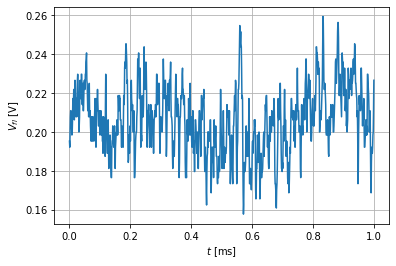At the time $t=5$ ms ($t=0$ ms is globally the time when the data acquisition system starts recording diagnostic signals) the discharge is procedure is initiated. Since the sampling frequency is 1 MHz (distance 1 $\mu$s between individual voltage samples), we average the voltage in the first 4000 samples (4 ms) to calculate the offset.

In :
offset = data[:1000].mean()
data = data - offset
pl.plot(t*1000, data)
pl.xlabel('$t$ [ms]')
pl.ylabel('$V_{fl}$ [V]')
pl.grid(True)
pl.xlim(t1*1000-2,t2*1000+2)

Out:
(2.2809999999999997, 15.736)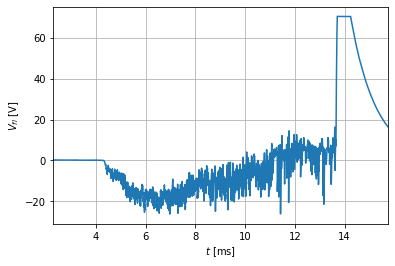## Electron temperature calculation¶

To measure the electron temperature $T_e$, both the ball-pen probe and the Langmuir probe must be so-called floating. This means that they are electrically insulated from their surroundings and we measure the voltage which the plasma particles will charge them to. This floating voltage is different for both probes due to their different design. The ball-pen probe floating voltage is

$V_{BPP} = \Phi - 0.6T_e$

where $\Phi$ is the plasma potential, and the Langmuir probe floating voltage is

$V_{fl} = \Phi - 2.8T_e$.

It then follows that the electron temperature can be calculated by subtracting the two floating voltages:

$T_e = \dfrac{V_{BPP}-V_{LP}}{2.2}.$

In the following, both the probe signals are loaded and visualised to show that the ball-pen probe floating voltage is, indeed, higher than the Langmuir probe floating voltage.

In :
# Load the signals
t, V_BPP = get_data('U_fl_BPP', shot)
t, V_fl = get_data('U_fl_LP', shot)

# Remove offsets
V_BPP -= V_BPP[:1000].mean()
V_fl -= V_fl[:1000].mean()

# Plot the signals
pl.plot(t*1000, V_fl, label='LP floating voltage')
pl.xlabel('$t$ [ms]')
pl.ylabel('$V_{fl}$ [V]')
pl.plot(t*1000, V_BPP, label='BPP floating voltage')
pl.xlabel('$t$ [ms]')
pl.ylabel('$V_{BPP}$ [V]')
pl.legend()
pl.grid(True)
pl.xlim(t1*1000-2,t2*1000+2)

Out:
(2.2809999999999997, 15.736)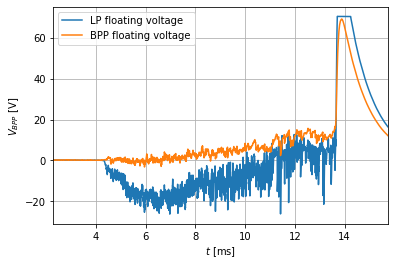We then calculate the electron temperature by subtracting the two signals.

In :
Te = (V_BPP-V_fl)/2.5
pl.plot(t*1000, Te, label='Electron temperature')
pl.xlabel('$t$ [ms]')
pl.ylabel('$T_e$ [eV]')
pl.legend()
pl.grid(True)
pl.xlim(t1*1000-2,t2*1000+2)
pl.savefig('icon-fig')